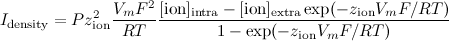GHK & Nernst examples
 Contributors

# Goldman-Hodgkin-Katz and Nernst formalisms in NeuroML2¶

Sample LEMS & NeuroML 2 files implementing a single compartment model, containing HH type Na+/K+ channels, a Ca++ channel and pool. The Calcium current through the channel can be calculated either through the GHK flux equation, or using the Nernst Equation to approximate its reversal potential.

The same mechanisms are implemented in NEURON as a comparison. Notice that NEURON has built in support for calculating reversal potentials via the Nernst equation, which can be compared to our implementation written from scratch by running Native NEURON , from scratch ; see also native Nernst modfile and from scratch modfile

## Running tests¶

The following tests can be run to compare the usage of GHK/Nernst from NEURON/LEMS:

``` git clone git@github.com:OpenSourceBrain/ghk-nernst.git
cd ghk-nernst/NEURON/
nrnivmodl
./ghk_na_k_ca.py
./nernst_na_k_ca.py

cd ../NeuroML2
jnml LEMS_ghk_na_k_ca.xml
jnml LEMS_nernst_na_k_ca.xml

cd ../
python compare.py
```

## neuroConstruct¶

Recent versions of neuroConstruct support GHK, generating NeuroML2 and NEURON code accordingly. In order to use the GHK flux equation instead
of Ohm’s law for a particular mechanism, set an extra parameter GHK_permeability (in m/s) using the Edit Density Mechs dialog.## Definitions¶

From WikipediaFrom Steuber et al DCN model:

``` A = exp(-23.20764929 * v / T) = exp((-z * F * (0.001) * v) / (R * T))

ical = perm * m*m * h * (4.47814e6 * v / T) * ((cali/1000) - (calo/1000) * A) / (1 - A)

(z^2 * F^2 * (0.001) * v) / (R * T)
```

From GENESIS

```          valency * F
K     =  - - - - - - -
R * (T + 273)

Cin * exp(K * Vm) - Cout
Ik    =  -p * F * K * Vm  - - - - - - - - - - - -
exp(K * Vm) - 1

(it is  missing one valency factor in comparison to the others, check genesis source)
```